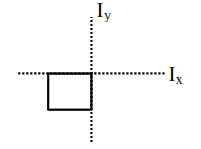# Moment of inertiaQuestion:

Moment of inertia of a square plate of side $l$ about the axis passing through one of the corner and perpendicular to the plane of square plate is given by :

1. $\frac{\mathrm{M} l^{2}}{6}$

2. $\mathrm{M} l^{2}$

3. $\frac{\mathrm{M} l^{2}}{12}$

4. $\frac{2}{3} \mathrm{M} l^{2}$

Correct Option: , 4

Solution:

According to perpendicular Axis theorem.$\mathrm{I}_{\mathrm{x}}+\mathrm{I}_{\mathrm{y}}=\mathrm{I}_{\mathrm{z}}$

$\mathrm{I}_{\mathrm{z}} \Rightarrow \frac{\mathrm{m} \ell^{2}}{3}+\frac{\mathrm{m} \ell^{2}}{3}$

$=\frac{2 m \ell^{2}}{3}$